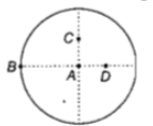Two spheres A and B of masses  respectively collide. A is at rest initially and B is moving with velocity v along x-axis. After collision B has a velocity $\frac{v}{2}$ in a direction perpendicular to the original direction.The mass A moves after collision in the direction

(a)same as that of B

(b)opposite to that of B

(c)

(d)

Concept Questions :-

Linear momentum
High Yielding Test Series + Question Bank - NEET 2020

Difficulty Level:

The moment of inertia of a uniform circular

disc is maximum about an axis perpendicular

to the disc and passing through(a) B                    (b) C

(c) D                    (d) A

Concept Questions :-

Moment of inertia
High Yielding Test Series + Question Bank - NEET 2020

Difficulty Level:

Three masses are placed on the x-axis:

300 g at origin, 500 g at x= 40 cm and 400g

at x=70 cm. The distance of the center of

mass from the origin is

(a) 40 cm                   (b) 45 cm

(c) 50 cm                   (d) 30 cm

Concept Questions :-

Center of mass
High Yielding Test Series + Question Bank - NEET 2020

Difficulty Level:

The instantaneous angular position of a point on

a rotating wheel is given by the equation

$\mathrm{\theta }\left(\mathrm{t}\right)=2{\mathrm{t}}^{3}-6{\mathrm{t}}^{2}$

The torque on the wheel becomes zero at

(a) t=0.5 s

(b) t=0.25 s

(c) t=2 s

(d) t=1 s

Concept Questions :-

Torque
High Yielding Test Series + Question Bank - NEET 2020

Difficulty Level:

The moment of inertia of a thin uniform rod of

mass M and length L about an axis passing

through its mid-point and perpendicular to its

length is ${\mathrm{I}}_{0}$. Its moment of inertia about an

axis passing through one of its ends and

perpendicular to its length is

(a) ${\mathrm{I}}_{0}+{\mathrm{ML}}^{2}/4$

(b) ${\mathrm{I}}_{0}+2{\mathrm{ML}}^{2}$

(c) ${\mathrm{I}}_{0}+{\mathrm{ML}}^{2}$

(d) ${\mathrm{I}}_{0}+{\mathrm{ML}}^{2}/2$

Concept Questions :-

Moment of inertia
High Yielding Test Series + Question Bank - NEET 2020

Difficulty Level:

A body of mass M hits normally a rigid wall with velocity v and bounces back with the same velocity. The impulse experienced by the body is

(a) 1.5 Mv                                                 (b) 2 Mv

(c) zero                                                     (d) Mv

Concept Questions :-

Linear momentum
High Yielding Test Series + Question Bank - NEET 2020

Difficulty Level:

A mass m moving horizontally (along the x-axis) with velocity v collides and sticks to mass of 3m moving vertically upward (along the y-axis) with velocity 2v. The final velocity of the combination is

(a) $\frac{1}{4}\mathrm{v}\stackrel{\mathbf{^}}{\mathbf{i}}+\frac{3}{2}\mathrm{v}\stackrel{\mathbf{^}}{\mathbf{j}}$                                   (b) $\frac{1}{3}\mathrm{v}\stackrel{\mathbf{^}}{\mathbf{i}}+\frac{2}{3}\mathrm{v}\stackrel{\mathbf{^}}{\mathbf{j}}$

(c) $\frac{2}{3}\mathrm{v}\stackrel{\mathbf{^}}{\mathbf{i}}+\frac{1}{3}\mathrm{v}\stackrel{\mathbf{^}}{\mathbf{j}}$                                    (d) $\frac{3}{2}\mathrm{v}\stackrel{\mathbf{^}}{\mathbf{i}}+\frac{1}{4}\mathrm{v}\stackrel{\mathbf{^}}{\mathbf{j}}$

High Yielding Test Series + Question Bank - NEET 2020

Difficulty Level:

A circular disk of moment of inertia ${I}_{t}$ is rotating in a horizontal plane, about its symmetry axis, with a constant angular speed ${\omega }_{i}.$ Another disk of moment of inertia ${I}_{b}$ is dropped coaxially onto the rotation disk. Initially the second disk has zero angular speed. Eventually both the disks rotate with a constant angular speed ${\omega }_{f}.$ The energy lost by the initially rotating disc to friction is

(a)$\frac{1}{2}\frac{{I}_{b}^{2}}{\left({I}_{t}+{I}_{b}\right)}{\omega }_{i}^{2}$                                     (b)$\frac{1}{2}\frac{{I}_{t}^{2}}{\left({I}_{t}+{I}_{b}\right)}{\omega }_{i}^{2}$

(c)$\frac{1}{2}\frac{{I}_{b}-{I}_{t}}{\left({I}_{t}+{I}_{b}\right)}{\omega }_{i}^{2}$                                     (d)$\frac{1}{2}\frac{{I}_{b}{I}_{t}}{\left({I}_{t}+{I}_{b}\right)}{\omega }_{i}^{2}$

Concept Questions :-

Angular momentum
High Yielding Test Series + Question Bank - NEET 2020

Difficulty Level:

Two particles which are initially at rest, move towards each other under the action of their mutual attraction.If their speeds are v and 2v at any instant, then the speed of centre of mass of the system will be

1. 2v                                             2. 0

3. 1.5v                                          4. v

Concept Questions :-

Center of mass
High Yielding Test Series + Question Bank - NEET 2020

Difficulty Level:

A man of 50 kg mass is standing in a gravity free space at a height of 10m above the floor. He throws a stone of 0.5 kg mass downwards with a speed  When the stone reaches the floor, the distance of the man above the floor will be

(a) 9.9m                                 (b) 10.1m

(c) 10m                                  (d) 20m

Concept Questions :-

Center of mass